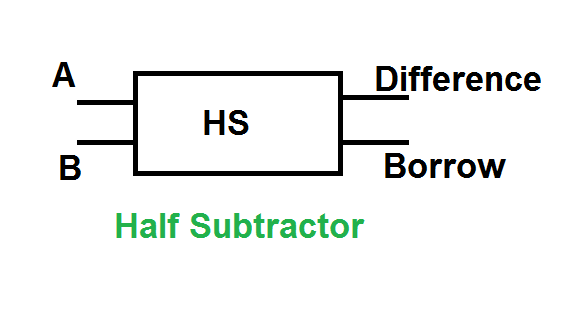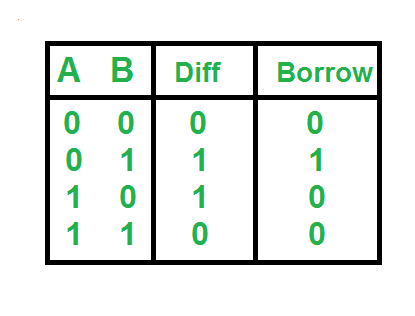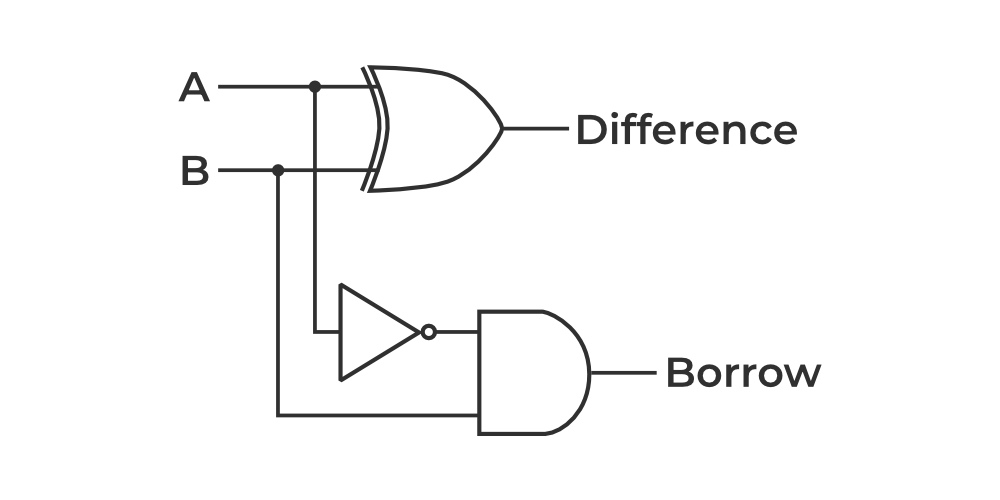Open In App
Related Articles

# Half Subtractor in Digital Logic

A half subtractor is a digital logic circuit that performs binary subtraction of two single-bit binary numbers. It has two inputs, A and B, and two outputs, DIFFERENCE and BORROW. The DIFFERENCE output is the difference between the two input bits, while the BORROW output indicates whether borrowing was necessary during the subtraction.

The half subtractor can be implemented using basic gates such as XOR and NOT gates. The DIFFERENCE output is the XOR of the two inputs A and B, while the BORROW output is the NOT of input A and the AND of inputs A and B.

## Half Subtractor

Half subtractor is a combination circuit with two inputs and two outputs that are different and borrow. It produces the difference between the two binary bits at the input and also produces an output (Borrow) to indicate if a 1 has been borrowed. In the subtraction (A-B), A is called a Minuend bit and B is called a Subtrahend bit.Truth TableThe SOP form of the Diff and Borrow is as follows:

Diff= A'B+AB'
Borrow = A'B

ImplementationLogical Expression

Difference = A XOR B
Borrow = \overline{A}B

1. Simplicity: The half adder and half subtractor circuits are simple and easy to design, implement, and debug compared to other binary arithmetic circuits.
2. Building blocks: The half adder and half subtractor are basic building blocks that can be used to construct more complex arithmetic circuits, such as full adders and subtractors, multiple-bit adders and subtractors, and carry look-ahead adders.
3. Low cost: The half adder and half subtractor circuits use only a few gates, which reduces the cost and power consumption compared to more complex circuits.
4. Easy integration: The half adder and half subtractor can be easily integrated with other digital circuits and systems.

1. Limited functionality: The half adder and half subtractor can only perform binary addition and subtraction of two single-bit numbers, respectively, and are not suitable for more complex arithmetic operations.
2. Inefficient for multi-bit numbers: For multi-bit numbers, multiple half adders or half subtractors need to be cascaded, which increases the complexity and decreases the efficiency of the circuit.
3. High propagation delay: The propagation delay of the half adder and half subtractor is higher compared to other arithmetic circuits, which can affect the overall performance of the system.

Application of Half Subtractor in Digital Logic:

1.Calculators: Most mini-computers utilize advanced rationale circuits to perform numerical tasks. A Half Subtractor can be utilized in a number cruncher to deduct two parallel digits from one another.

2.Alarm Frameworks: Many caution frameworks utilize computerized rationale circuits to identify and answer interlopers. A Half Subtractor can be utilized in these frameworks to look at the upsides of two parallel pieces and trigger a caution in the event that they are unique.

3.Automotive Frameworks: Numerous advanced vehicles utilize computerized rationale circuits to control different capabilities, like the motor administration framework, stopping mechanism, and theater setup. A Half Subtractor can be utilized in these frameworks to perform computations and examinations.

4.Security Frameworks: Advanced rationale circuits are usually utilized in security frameworks to identify and answer dangers. A Half Subtractor can be utilized in these frameworks to look at two double qualities and trigger a caution in the event that they are unique.

5.Computer Frameworks: Advanced rationale circuits are utilized broadly in PC frameworks to perform estimations and examinations. A Half Subtractor can be utilized in a PC framework to deduct two paired values from one another.